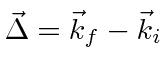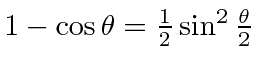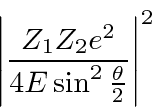## Scattering from a Screened Coulomb Potential

A standard Born approximation example is Rutherford Scattering, that is, Coulomb scattering of a particle of chargein a screened Coulomb potential. The exponential represents the screening of the nuclear charge by atomic electrons. Without screening, the total Coulomb scattering cross section is infinite because the range of the force in infinite.

The potential energy then isWe need to calculate its Fourier Transform.Since the potential has spherical symmetry, we can chooseto be in thedirection and proceed with the integral.Since, we have. For elastic scattering,. The differential cross section isIn the last step we have used the non-relativistic formula for energy and.

The screened Coulomb potential gives a finite total cross section. It corresponds well with the experiment Rutherford did in whichparticles were scattered from atoms in a foil. If we scatter from a bare charge where there is no screening, we can take the limit in which.The total cross section diverges in due to the region around zero scattering angle.

Jim Branson 2013-04-22